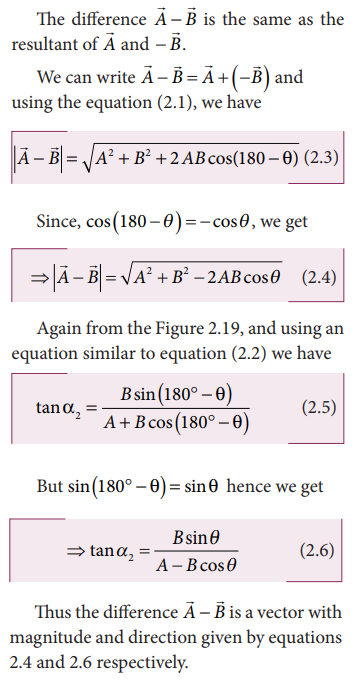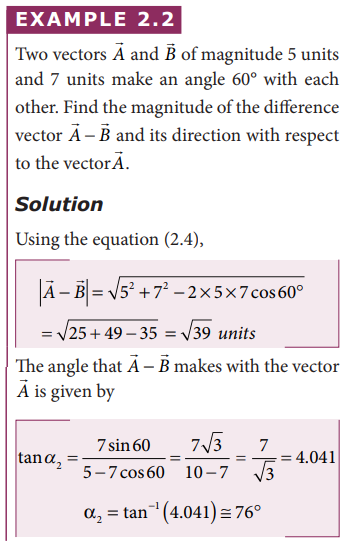Home | | Physics 11th std | Subtraction of vectors

# Subtraction of vectors

Since vectors have both magnitude and direction two vectors cannot be subtracted from each other by the method of ordinary algebra.

Subtraction of vectors

Since vectors have both magnitude and direction two vectors cannot be subtracted from each other by the method of ordinary algebra. Thus, this subtraction can be done either geometrically or analytically. We shall now discuss subtraction of two vectors geometrically using the Figure 2.19

For two non-zero vectorsandwhich are inclined to each other at an angle θ, the differenceis obtained as follows. First obtainas in Figure 2.19. The angle betweenandis 180-θ.## Solved Example Problem for Subtraction of vectorsTags : with Solved Example Problems , 11th Physics : UNIT 2 : Kinematics
Study Material, Lecturing Notes, Assignment, Reference, Wiki description explanation, brief detail
11th Physics : UNIT 2 : Kinematics : Subtraction of vectors | with Solved Example Problems